Canonical correlation coefficients

Maximum values of correlation coefficients between pairs of linear functions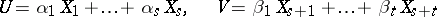of two sets of random variables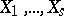and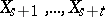for which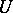andare canonical random variables (see Canonical correlation). The problem of determining the maximum correlation coefficient betweenandunder the conditionsand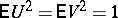can be solved using Lagrange multipliers. The canonical correlation coefficients are the roots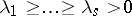of the equationwhere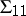and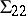are the covariance matrices ofand, respectively, and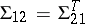is the covariance matrix between the variables of the first and second sets. The-th root of the equation is called the-th canonical correlation coefficient betweenand. It is equal to the maximum value of the correlation coefficients between the pair of linear functionsand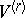of canonical random variables, each of which has variance one and is uncorrelated with the first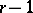pairs of variablesand. The coefficients,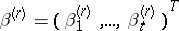of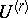andsatisfy the equation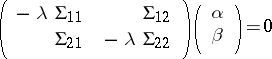when.